# Linear Discriminant Analysis¶

Linear Discriminant Analysis are statistical analysis methods to find a linear combination of features for separating observations in two classes.

Note: Please refer to Multi-class Linear Discriminant Analysis for methods that can discriminate between multiple classes.

## Overview of LDA¶

Suppose the samples in the positive and negative classes respectively with means: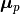and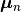, and covariances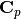and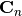. Then based on Fisher’s Linear Discriminant Criteria, the optimal projection direction can be expressed as: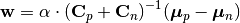Here α is an arbitrary non-negative coefficient.

## Linear Discriminant¶

A linear discriminant functional can be written as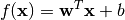Here, w is the coefficient vector, and b is the bias constant.

This package uses the LinearDiscriminant type, defined as below, to capture a linear discriminant functional:

immutable LinearDiscriminant <: Discriminant
w::Vector{Float64}
b::Float64
end


This type comes with several methods. Let f be an instance of LinearDiscriminant

length(f)

Get the length of the coefficient vector.

evaluate(f, x)

Evaluate the linear discriminant value, i.e w'x + b.

When x is a vector, it returns a real value; when x is a matrix with samples in columns, it returns a vector of length size(x, 2).

predict(f, x)

Make prediction. It returns true iff evaluate(f, x) is positive.

## Data Analysis¶

The package provides several functions to perform Linear Discriminant Analysis.

ldacov(Cp, Cn, μp, μn)

Performs LDA given covariances and mean vectors.

Parameters: Cp – The covariance matrix of the positive class. Cn – The covariance matrix of the negative class. μp – The mean vector of the positive class. μn – The mean vector of the negative class. The resultant linear discriminant functional of type LinearDiscriminant.

Note: The coefficient vector is scaled such that w'μp + b = 1 and w'μn + b = -1.

ldacov(C, μp, μn)

Performs LDA given a covariance matrix and both mean vectors.

Parameters: C – The pooled covariane matrix (i.e (Cp + Cn)/2) μp – The mean vector of the positive class. μn – The mean vector of the negative class. The resultant linear discriminant functional of type LinearDiscriminant.

Note: The coefficient vector is scaled such that w'μp + b = 1 and w'μn + b = -1.

fit(LinearDiscriminant, Xp, Xn)

Performs LDA given both positive and negative samples.

Parameters: Xp – The sample matrix of the positive class. Xn – The sample matrix of the negative class. The resultant linear discriminant functional of type LinearDiscriminant.